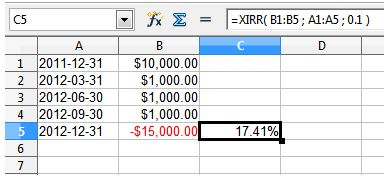Share:

One needs to ask how much returns does one get by investing in any form. Investment could be in any form short term or long term, but we are always curious to know how much are we making money out of money! And so for this we have various methods to calculate investment returns.

## Calculating returns  using IRR(Internal Rate of Return) Function

### What is IRR?

Internal rate of return is a discount rate that makes the net present value (NPV) of all cash flows from a particular project equal to zero. In short IRR is the rate of growth your investment will generate.It is very easy to calculate IRR and this can be done with the help of Excel formula as shown below:

=IRR(values,[guess])

The terms  are explained as follows:

‘values’ refer to the amount that has been either invested in or redeemed from the fund

‘guess’ is a number that you select is close to the result of IRR.

Now here one assumption is made that the investments in values field is periodical and so only values are considered while calculating the formula.

### Drawbacks of IRR

Since IRR considers periodic investments to be made in the funds or redeemed, is quite inconvenient to calculate the real world scenarios. Because in reality we invest or redeem money from the funds based on our convenience. Hence a more appropriate method of calculating it that is XIRR is used for calculating returns.

## Calculating returns using XIRR function

### What is XIRR?

XIRR(Extended Internal Rate of Return) function is similar to IRR but doesn’t consider that we do regular investments like IRR and hence it is more robust.

The syntax for using XIRR is

XIRR(values, dates, [guess])

The terms  are explained as follows:

‘values’ are the range of portfolio values; ‘dates’ are the range of dates; and ‘guess’ is an approximate percentage return (optional).

### Requirements for calculating XIRR:

1. SIP amount
2. Dates of SIP investments
3. Date of redemption, and
4. Maturity (redemption) amount

Consider the following example for calculating IRR:In the example as we see , at the end of 2011, the portfolio value was \$10,000. Throughout 2012, there were 3 contributions to the account at various times for which the amount is as shown that is  \$1,000.

The final value at the end of 2012 was \$15,000. It is negative since we have invested in the fund and the money is taken out from our pockets. Using the XIRR function, the return for this portfolio is 17.41%.

The formula can be seen in the titlebar of the screen shot and is as per the one mentioned above.

So to summarize in this article we have seen how to calculate returns for investments in mutual funds through two methods namely IRR that is Internal Rate of Return and XIRR that is Extended Internal Rate of Return, each functions pros and cons and uses for calculating returns.

Investing in mutual funds is now as easy as investing online. Log on to Groww.

Disclaimer: the views expressed here are those of the author. Mutual funds are subject to market risks. Please read the offer document before investing.

Share: